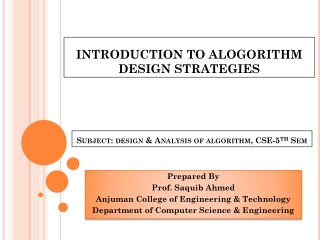DownloadDownload PresentationINTRODUCTION TO ALOGORITHM DESIGN STRATEGIES

# INTRODUCTION TO ALOGORITHM DESIGN STRATEGIES

Télécharger la présentation## INTRODUCTION TO ALOGORITHM DESIGN STRATEGIES

- - - - - - - - - - - - - - - - - - - - - - - - - - - E N D - - - - - - - - - - - - - - - - - - - - - - - - - - -
##### Presentation Transcript

1. INTRODUCTION TO ALOGORITHM DESIGN STRATEGIES Subject: design & Analysis of algorithm, CSE-5thSem Prepared By Prof. Saquib Ahmed Anjuman College of Engineering & Technology Department of Computer Science & Engineering

2. Syllabus UNIT-I Mathematical foundations, summation of arithmetic and geometric series, n, n2 , bounding summations using integration, Recursion and Induction: recurrence relations, solutions of recurrence relations using techniques of characteristic equation, generating functions, master method and substitution method.Complexity calculation of various standard functions, principles of designing algorithms. UNIT-II Asymptotic notations of analysis of algorithms, analyzing control structures, worst case and average case analysis, amortized analysis, application of amortized analysis, Sorting networks, comparison networks, bio-tonic sorting network, advanced data structures like Fibonacci heap, disjoint set representation UNIT-III Divide and conquer basic strategy, binary search, quick sort, merge sort, matrix operations, Multiplication Algorithm Greedy method – basic strategy, Knapsack Problem, application to job sequencing with deadlines problem, minimum cost spanning trees, single source shortest path, Optimal Search Patterns. UNIT-IV Dynamic Programming basic strategy, multistage graphs, all pairs shortest path, single source shortest paths, optimal binary search trees, traveling salesman problem, Longest Common Subsequence problem, 0/1 Knapsack Problem, Chained Matrix Multiplication UNIT-V Basic Traversal and Search Techniques, breadth first search and depth first search, connected components. Backtracking basic strategy, 8-Queen’s problem, graph coloring, Hamiltonian cycles etc, Introduction to Approximation algorithm. UNIT-VI NP-hard and NP-complete problems, basic concepts, non-deterministic algorithms, NP-hard and NP-complete, decision and optimization problems, graph based problems on NP Principle

3. COUURSE OUTCOMES

4. CONTENTS Strategies to Algorithm Design: • Greedy Method • Divide and Conquer Method • Dynamic Programming Method

5. Greedy algorithms • Greedy algorithms are short sighted algorithms • Decision is based upon incomplete information. • The decision are made without bothering about its effect on later part of generated information. • Greedy algorithms are most efficient algorithms if executed. • Examples of Greedy Algorithms are Knapsack, Minimum Cost Spanning Trees, Single Source Shortest Path (SSSP) algorithms.

6. Greedy algorithms are most efficient algorithms if executed.. JUSTIFY B 5 10 Destination Source A D 10 C 2 Figure 1: Greedy Algorithm Decision

7. JUSTIFICATION • As seen in figure 1, since the information generated is not stored as well as no time is consumed in making the decision. • Therefore execution speed of algorithm will be much higher. • Due to this efficiency increases, hence statement is true.

8. Divide and conquer algorithms DIVIDE AND CONQUER ALGORITHMS • In this method, the given problem is divided into sub-problems till the sub-problem is easily solvable • The decision is continued until the sub-problems are solvable. • Each sub-problem is solved using the same principle • For every sub-problem there will be a single solution • Hence, in Divide and Conquer (DAC) the size of the input is reduced so as to reduce the size of a problem. P p1 p2 p3 p4

9. Dynamic programming algorithms • Dynamic Programming operates on principle of Optimality • Assume that the path between A & B is optimal. In this Path, there is another path X-Y. • Since the path between A-B is optimal, therefore path X-Y is also optimal. Shortest Path A B Optimal Path A X Y B Optimal Path

10. Dynamic programming algorithms • In Dynamic Programming strategy, the decision making is delayed till all the information is available. • This will require larger storage as well as the complexity of decision making process will increase.

11. THANK YOU